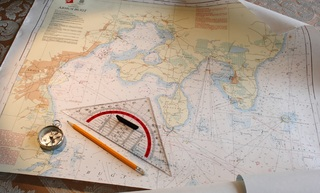Latitude/Longitude DMS to Decimal Degrees Calculator
Latitude/Longitude DMS to Decimal Degrees Calculator
##### Instructions/Notes

This tool converts your latitude/longitude position from degrees, minutes, seconds (DMS) to decimal degrees format. Simply enter the DMS value of your position below.

For example, using 38° 53' 21.948", degrees is 38, minutes is 53, and seconds is 21.948. The decimal degrees equivalent is 38.88943

Note: Positive latitude values (1 to 90) represent locations in the northern hemisphere (north of the equator), whereas negative latitude values (1- to -90) are locations in the southern hemisphere (south of the equator).

Positive longitude values (1 to 180) are locations in the eastern hemisphere (east of prime meridian ), whereas negative values (-1 to -180) are locations in the western hemisphere (west of prime meridian ).

See hemisphere map from Encyclopedia Britannica  for an illustration.Try our Latitude/Longitude Distance Calculator to determine the distance between two points.

Try latitude 38° 53' 21.948" longitude -77° 2' 6.792"
Latitude:   Degrees:
Minutes:
Seconds:
Longitude:   Degrees:
Minutes:
Seconds: